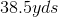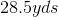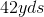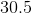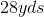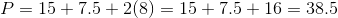# Intermediate Geometry : How to find the perimeter of a trapezoid

## Example Questions

### Example Question #1 : How To Find The Perimeter Of A Trapezoid

Find the perimeter of the following trapezoid.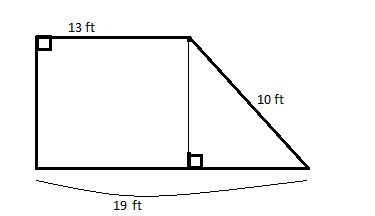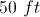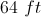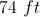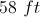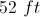Explanation:

The answer is 50 ft.  In order to find the perimeter, you must find the length of the left side.  After some deduction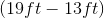, you can find that the base of the triangle is 6 ft.   Then using the Pythagorean Theorem, or 3-4-5 right triangles, you can find that the height of the triangle and rectangle is 8 ft.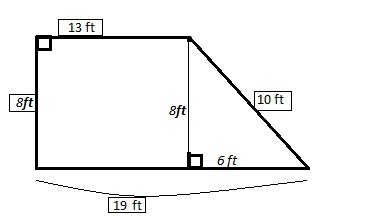Once you find that the last side is 8 ft, you can add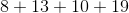to get an answer of 50 ft. for the perimeter.

### Example Question #2 : How To Find The Perimeter Of A Trapezoid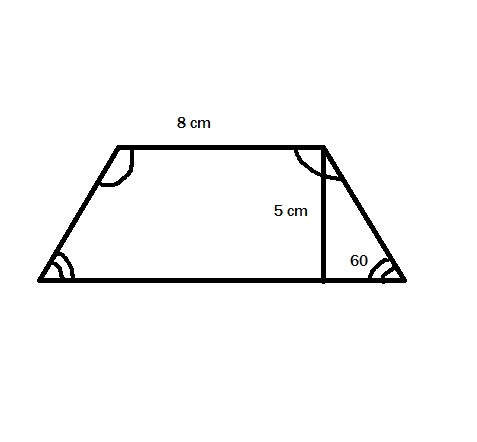The height of a trapezoid iscm and the length of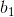iscm.

Find the perimeter of the trapezoid to the nearest hundreth.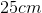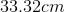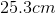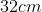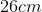Explanation:

Use the triangle formed by the height of the trapezoid to find the lengths of the two sides of the trapezoid and the length of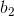: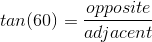.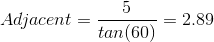cm.

This finds the base of the triangle, which can be added twice toto find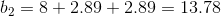cm.

Now, use the same triangle to find the length of the sides.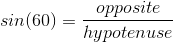.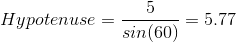cm.

Lastly, add all of the lengths together: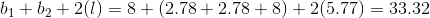cm.

### Example Question #3 : How To Find The Perimeter Of A Trapezoid

Find the perimeter of the trapezoid below.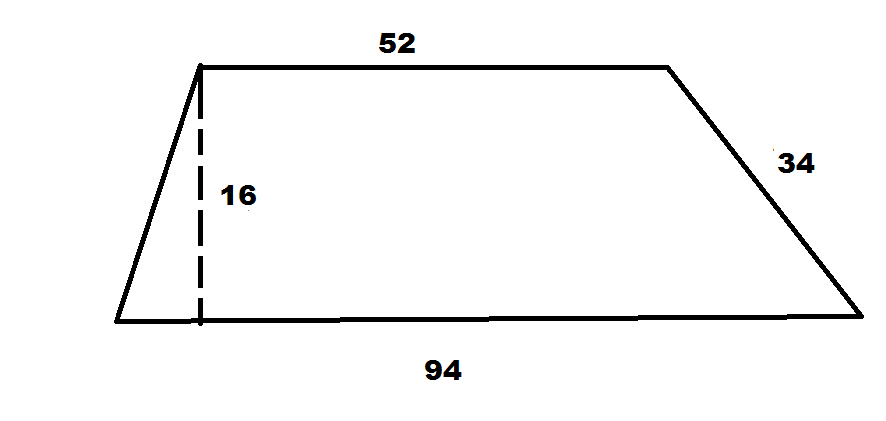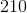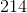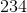Explanation: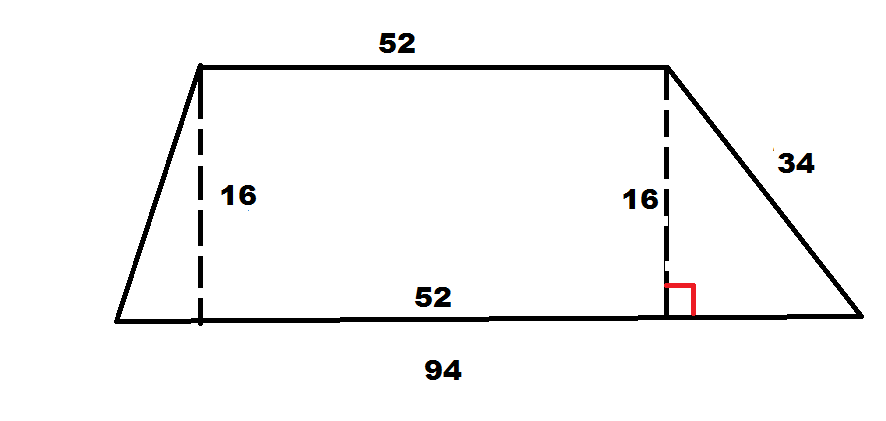We can then use the Pythagorean Theorem to find the right portion of the bottom base.  We can then use this value to determine the left portion.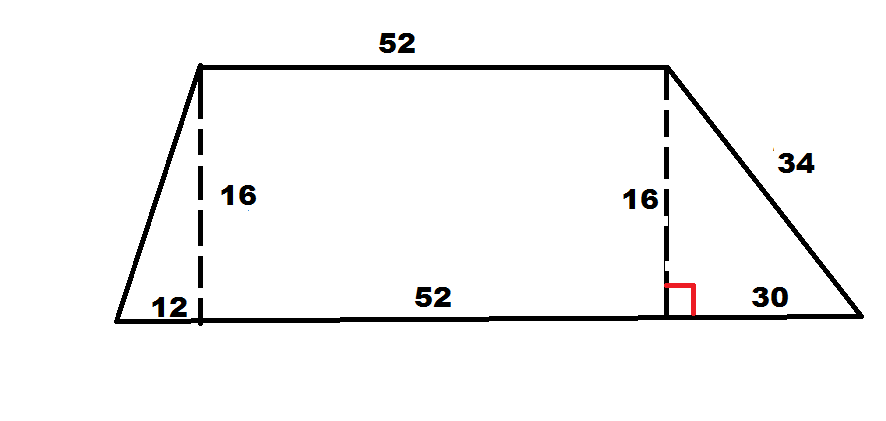Using Pythagorean Theorem again, we can calculate the left leg to be 20.  That means we now know all four sides.  The perimeter is simply the sum.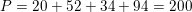### Example Question #1 : How To Find The Perimeter Of A Trapezoid

An isosceles trapezoid has two bases that are parallel to each other. The larger base istimes greater than the smaller base. The smaller base has a length ofinches and the length of non-parallel sides of the trapezoid have a length of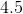inches.

What is the perimeter of the trapezoid?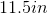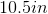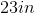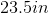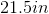Explanation:

To find the perimeter of this trapezoid, first find the length of the larger base. Then, find the sum of all of the sides. It's important to note that since this is an isosceles trapezoid, both of the non-parallel sides will have the same length.

The solution is:

The smaller base is equal toinches. Thus, the larger base is equal to: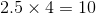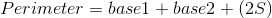, where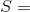the length of one of the non-parallel sides of the isosceles trapezoid.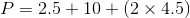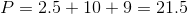### Example Question #5 : How To Find The Perimeter Of A Trapezoid

Dr. Robinson's property is shaped like an isosceles trapezoid. Dr. Robinson gave a contractor the following measurements, so that the contractor can build a wall around the enire property.

Measurements of property: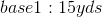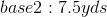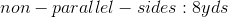Find the perimeter of Dr. Robinson's property.